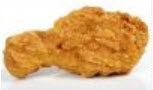# Random Variables: Mean, Variance and Standard Deviation

A Random Variable is a set of possible values from a random experiment.

### Example: Tossing a coin: we could get Heads or Tails.

Let's give them the values Heads=0 and Tails=1 and we have a Random Variable "X":So:

• We have an experiment (like tossing a coin)
• We give values to each event
• The set of values is a Random Variable

## Mean, Variance and Standard Deviation### Example: Tossing a single unfairdie

For fun, imagine a weighted die (cheating!) so we have these probabilities:

 1 2 3 4 5 6 0.1 0.1 0.1 0.1 0.1 0.5

### Mean or Expected Value: μ

When we know the probability p of every value x we can calculate the Expected Value (Mean) of X:

μ = Σxp

Note: Σ is Sigma Notation, and means to sum up.

To calculate the Expected Value:

• multiply each value by its probability
• sum them up### Example continued:

 x 1 2 3 4 5 6 p 0.1 0.1 0.1 0.1 0.1 0.5 xp 0.1 0.2 0.3 0.4 0.5 3

μ = Σxp = 0.1+0.2+0.3+0.4+0.5+3 = 4.5

The expected value is 4.5

Note: this is a weighted mean: values with higher probability have higher contribution to the mean.

### Variance: Var(X)

The Variance is:

Var(X) = Σx2p − μ2

To calculate the Variance:

• square each value and multiply by its probability
• sum them up and we get Σx2p
• then subtract the square of the Expected Value μ2### Example continued:

 x 1 2 3 4 5 6 p 0.1 0.1 0.1 0.1 0.1 0.5 x2p 0.1 0.4 0.9 1.6 2.5 18

Σx2p = 0.1+0.4+0.9+1.6+2.5+18 = 23.5

Var(X) = Σx2p − μ2 = 23.5 - 4.52 = 3.25

The variance is 3.25

### Standard Deviation: σ

The Standard Deviation is the square root of the Variance:

σ = √Var(X)### Example continued:

 x 1 2 3 4 5 6 p 0.1 0.1 0.1 0.1 0.1 0.5 x2p 0.1 0.4 0.9 1.6 2.5 18

σ = √Var(X) = √3.25 = 1.803...

The Standard Deviation is 1.803...

Let's have another example!

(Note that we run the table downwards instead of along this time.)### You plan to open a new McDougals Fried Chicken, and found these stats for similar restaurants:

Percent Year's Earnings
20% \$50,000 Loss
30% \$0
40% \$50,000 Profit
10% \$150,000 Profit

Using that as probabilities for your new restaurant's profit, what is the Expected Value and Standard Deviation?

The Random Variable is X = 'possible profit'.

Sum up xp and x2p:

Probability
p
Earnings (\$'000s)
x

xp

x2p
0.2 -50 -10 500
0.3 0 0 0
0.4 50 20 1000
0.1 150 15 2250
Σp = 1   Σxp = 25 Σx2p = 3750

μ = Σxp = 25

Var(X) = Σx2p − μ2
= 3750 − 252
= 3750 − 625
= 3125

σ = √3125 = 56 (to nearest whole number)

But remember these are in thousands of dollars, so:

• μ = \$25,000
• σ = \$56,000

So you might expect to make \$25,000, but with a very wide deviation possible.

Let's try that again, but with a much higher probability for \$50,000:

### Example (continued):

Now with different probabilities (the \$50,000 value has a high probability of 0.7 now):

Probability
p
Earnings (\$'000s)
x

xp

x2p
0.1 -50 -5 250
0.1 0 0 0
0.7 50 35 1750
0.1 150 15 2250
Σp = 1 Sums: Σxp = 45 Σx2p = 4250

μ = Σxp = 45

Var(X) = Σx2p − μ2
= 4250 − 452
= 4250 − 2025
= 2225

σ = √2225 = 47 (to nearest whole number)

In thousands of dollars:

• μ = \$45,000
• σ = \$47,000

The mean is now much closer to the most probable value.

And the standard deviation is a little smaller (showing that the values are more central.)

## Continuous

Random Variables can be either Discrete or Continuous:

• Discrete Data can only take certain values (such as 1,2,3,4,5)
• Continuous Data can take any value within a range (such as a person's height)

Here we looked only at discrete data, as finding the Mean, Variance and Standard Deviation of continuous data needs Integration.

## Summary

• A Random Variable is a variable whose possible values are numerical outcomes of a random experiment.
• The Mean (Expected Value) is: μ = Σxp
• The Variance is: Var(X) = Σx2p − μ2
• The Standard Deviation is: σ = √Var(X)